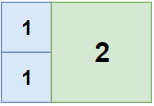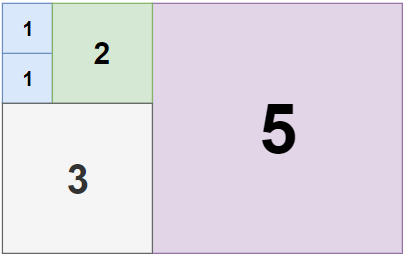Formatted question description: https://leetcode.ca/all/1240.html

# 1240. Tiling a Rectangle with the Fewest Squares

Hard

## Description

Given a rectangle of size n x m, find the minimum number of integer-sided squares that tile the rectangle.

Example 1:Input: n = 2, m = 3

Output: 3

Explanation: 3 squares are necessary to cover the rectangle.

2 (squares of 1x1)

1 (square of 2x2)

Example 2:Input: n = 5, m = 8

Output: 5

Example 3:Input: n = 11, m = 13

Output: 6

Constraints:

• 1 <= n <= 13
• 1 <= m <= 13

## Solution

Use dynamic programming. Create a 2D array dp of n + 1 rows and m + 1 columns, where dp[i][j] represents the minimum number of squares required to tile the i * j rectangle.

Obviously, if i == j, dp[i][j] = 1. Otherwise, the rectangle can be split by a horizontal line or a vertical line, which leads to two smaller rectangles, or a square may be put in the middle. For each case, calculate the minimum number of squares needed.

Finally, return dp[n][m].

class Solution {
public int tilingRectangle(int n, int m) {
int[][] dp = new int[n + 1][m + 1];
for (int i = 1; i <= n; i++) {
for (int j = 1; j <= m; j++) {
if (i == j)
dp[i][j] = 1;
else {
dp[i][j] = Integer.MAX_VALUE;
int rowStart = 1, rowEnd = i / 2;
for (int row = rowStart; row <= rowEnd; row++)
dp[i][j] = Math.min(dp[i][j], dp[row][j] + dp[i - row][j]);
int columnStart = 1, columnEnd = j / 2;
for (int column = columnStart; column <= columnEnd; column++)
dp[i][j] = Math.min(dp[i][j], dp[i][column] + dp[i][j - column]);
for (int row = 1; row <= i; row++) {
for (int column = 1; column <= j; column++) {
for (int side = Math.min(row, column) - 1; side > 0; side--)
dp[i][j] = Math.min(dp[i][j], 1 + dp[row - side][column] + dp[i - row + side][column - side] + dp[i - row][j - column + side] + dp[row][j - column]);
}
}
}
}
}
return dp[n][m];
}
}